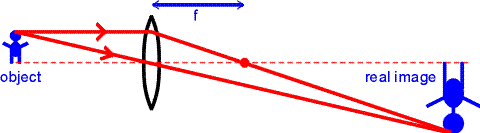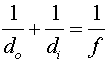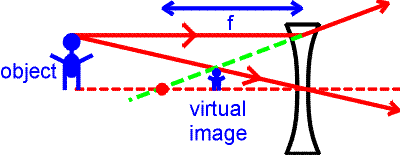Images, real and virtual Real images are those where light actually converges, whereas virtual images are locations from where light appears to have converged. Real images only occur when for objects which are placed outside the focal length of a convex lens. A real image is illustrated below. Ray tracing gives the position of the images by drawing one ray perpendicular to the lens that passes through the focal point, and a second ray that passes through the center of the lens (this ray is not bent by the lens). The intersection of the two rays gives the position of the image. Note that the real image is inverted and larger than the object.The position of the image can be found through the equation:Here, the distances are those of the object and image respectively as measured from the lens. The focal length f is positive for a convex lens. A positive image distance corresponds to a real image, just as it did for the case of the mirrors. However, for a lens, a positive image distance implies that the image is located on the opposite side as the object. Virtual images are formed by concave lenses or by placing an object inside the focal length of a convex lens. The ray-tracing exercise is repeated for the case of a virtual image.In this case the virtual image is upright and shrunken. The same formula for the image and object distances used above applies again here. Only in this case the focal length is negative, and the solution for the image distance will also be negative. Virtual images can also be produced by convex lenses when the object is placed inside the focal length. In that case, the virtual image will be upright and enlarged, as it will be further from the lens than the object.